# Scaling Composed Unit Ratios In Tandem

Build flexibility with visual math talk prompts involving scaling composed unit ratios in tandem on a double number line.

## In This Set of Math Visual Prompts…

Students will explore equivalent ratios and reveal rates through partitive division.

## Intentionality…

This set of visual math talk prompts is taken from the Math Talk section of Day 2 in the Make Math Moments Problem Based Unit called Rice Ratios. The purpose of the Math Talk is to reinforce key concepts and big ideas from the first day of this problem based math unit including:

• There are two types of ratios; composed unit and multiplicative comparison;
• A composed unit is often (not always) a ratio with two distinct units;
• A composed unit can be scaled in tandem;
• Equivalent ratios are derived from the same rate;
• When you divide a composed unit through partitive division, you reveal a rate.

Unsure about some of the terminology listed above?

## Preparing to Facilitate

In today’s visual math talk, we will be presenting the following composed unit to students:

200 ml of water : 250 g of sugar

Share the following quantities of water and sugar, one at a time. For each quantity, students must determine the appropriate amount of sugar or water based on the lemonade recipe above. In each scenario, encourage students to share their strategy and justify their thinking through the use of a model.

The three (3) visual math talk prompts we will share here include:

100 ml of water : ? g of sugar

? ml of water : 500 g of sugar

40 ml of water : ? g of sugar

An additional three (3) prompts are shared in the Teacher Guide from Day 2 of the Rice Ratios problem based math unit

You might consider tracking the equivalent ratios in a ratio table as well as leveraging a handful of visual math talk prompts such as the following silent solution animations shared below.

## Problem #1

The first problem in the visual math talk prompt asks students to determine how much sugar should be used with 100 ml of water.

Since we are dealing with a composed unit ratio comparing the quantity of water in millilitres and the quantity of sugar in grams required for a lemonade recipe, students are encouraged to leverage the double number line. The bars that are shared in the silent solution video will help students to scale in tandem to determine other equivalent ratios from this ratio relationship.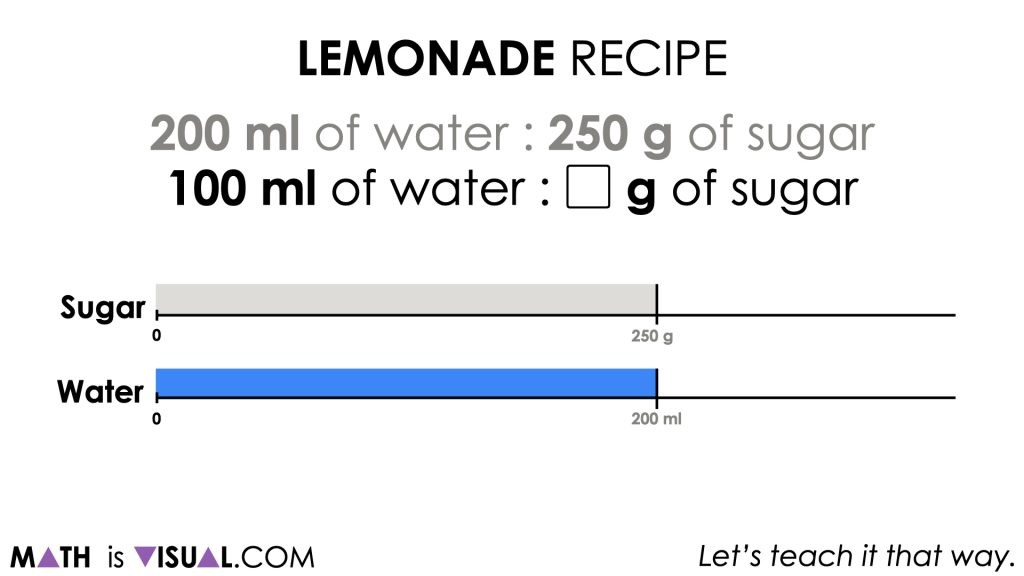By providing students with a visual mathematical model, they will likely be able to utilize their spatial reasoning and understanding of magnitude of number to label 100 ml of water at the halfway point between 0 and 200.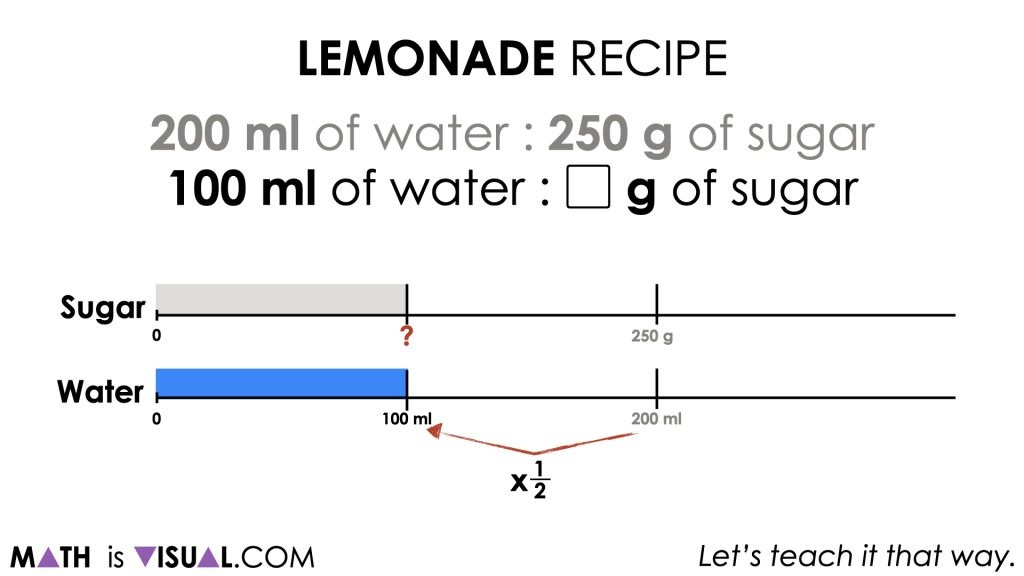They then can leverage their understanding of halving or other strategies to determine the amount of sugar that will ensure the ratio equivalence is maintained.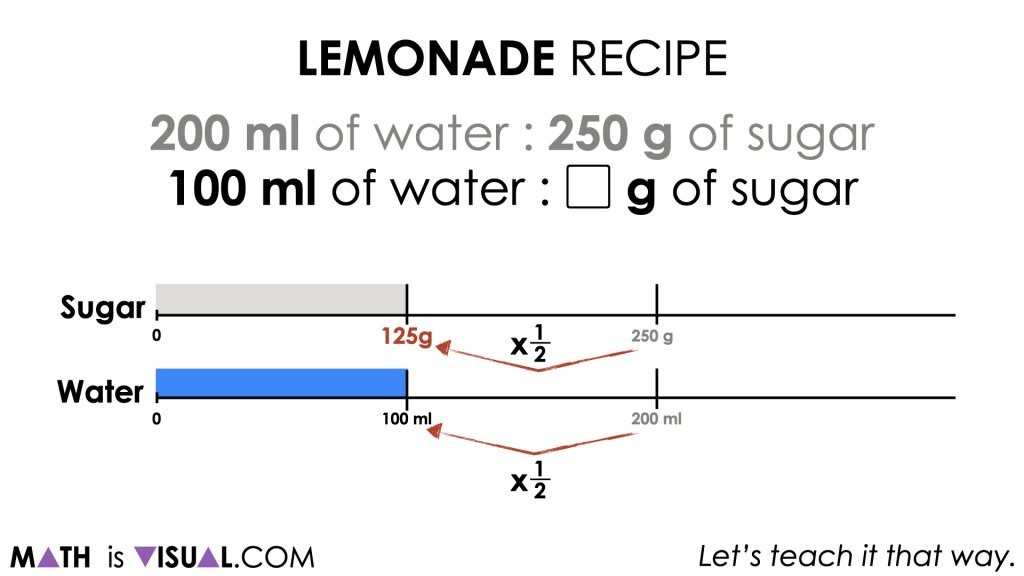Therefore, 125 g of sugar is required to satisfy the recipe if 100 ml of water is used.

## Problem #2:

In this second prompt, students will leverage the same mathematical model to determine the amount of water required to complete the recipe if 500 g of sugar is used.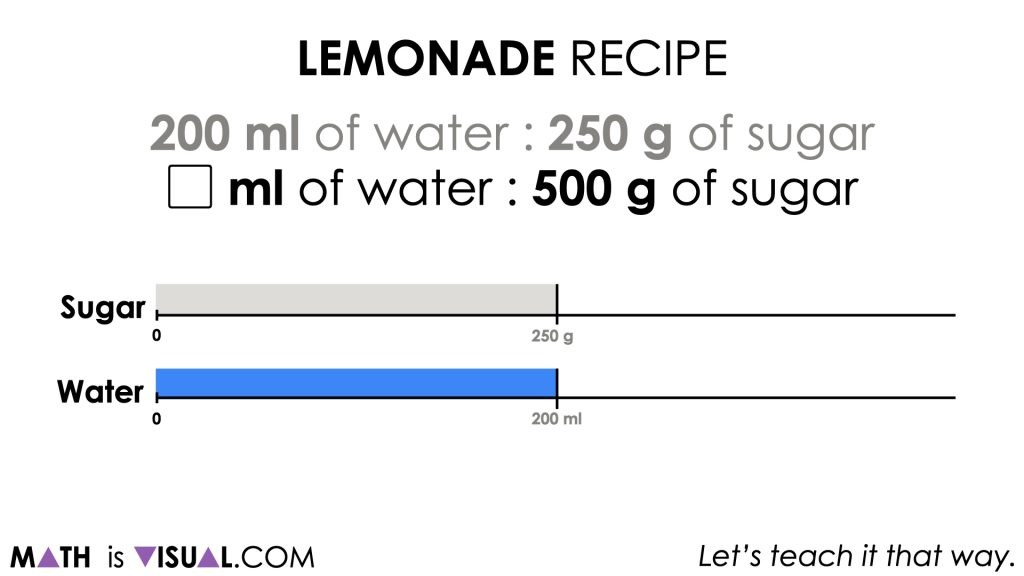Observe how students determine the amount of water required. Are they using a skip counting method to guess and check? Are they thinking additively? Is 500 g divided by 250 g a known fact?

This thinking can help you determine what strategies students are bringing to the problem and where you might want to spend more time.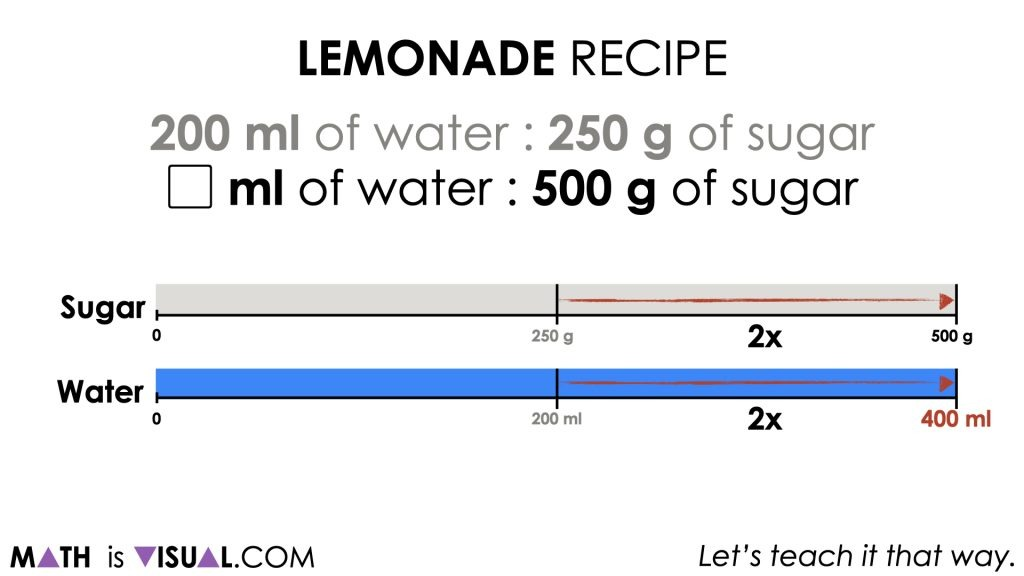## Problem #3:

The 3rd problem in this visual math talk asks how much sugar should be used with 40 ml of water.

This prompt pushes student thinking a little further requiring them to determine what fraction 40 ml is compared to 200 ml.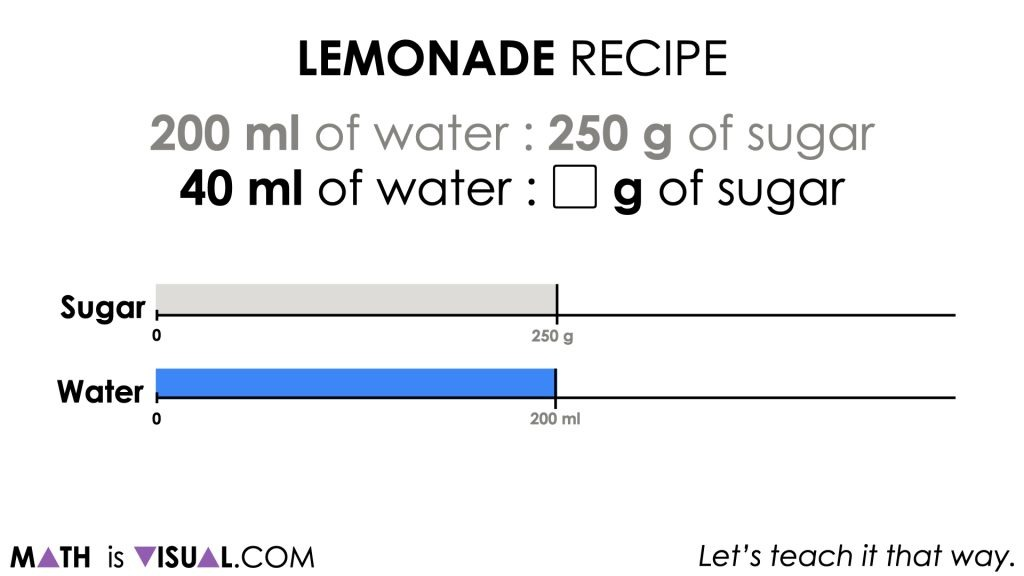Observe how students work through this problem.

Are they able to place 40 ml fairly accurately on the number line or is there some work to be done with magnitude of number?

In order for students to flexibly navigate through this problem, they will need to convince themselves that 40 ml is 1 fifth of the way between 0 and 200. Ask students to convince you and take note of the strategy they use to do this.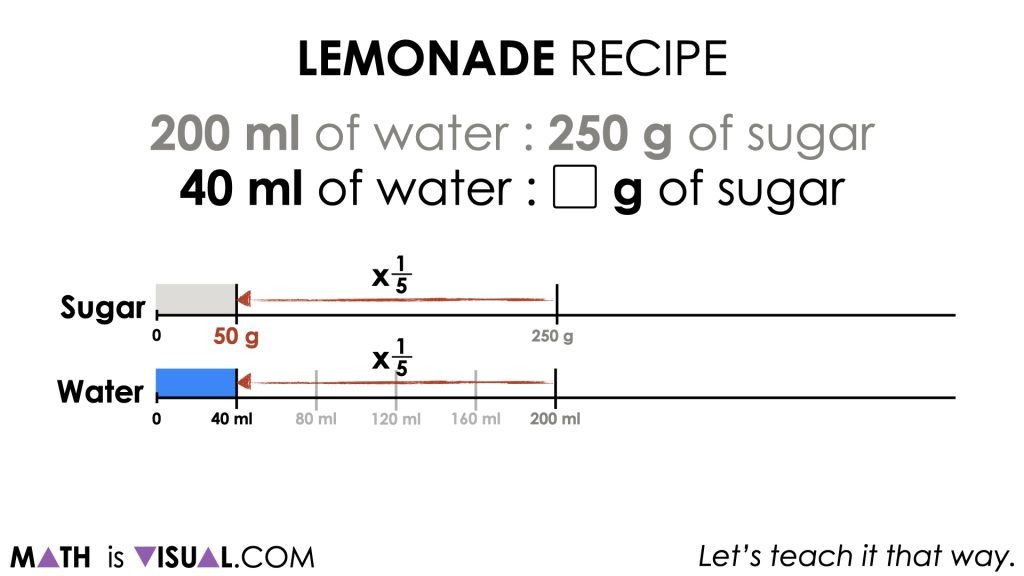Since 40 ml is 1 fifth of 200 ml, then we can determine the amount of sugar needed by taking 1 fifth of 250 g resulting in a total of 50 g of sugar.

## Want to Explore These Concepts & Skills Further?

Three additional math talk prompts are available in Day 2 of the Rice Ratios problem based math unit that you can dive into now.

Why not start from the beginning of this contextual 5-day unit of ratios and rates real world lessons from the Make Math Moments Problem Based Units page.

Did you use this in your classroom or at home? How’d it go? Post in the comments!

Math IS Visual. Let’s teach it that way.